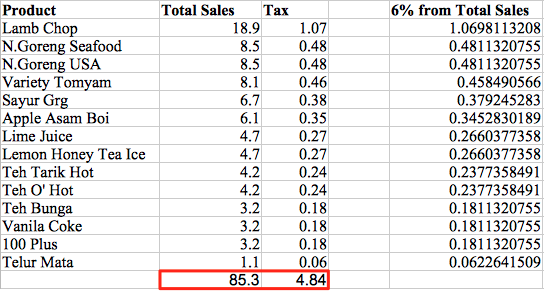When doing a transaction, the system will store the tax of every item in that transaction up to 2 rounding decimals. The calculation of total tax for a day would be summing up all the tax that the system stored on each item. So the sum up amount will be a little bit different than the amount that you extracted 6% from total amount(To get the 6% from the Total Amount we'll have to use this formula Total Amount - Total Amount / 1.06).

Example of a Daily Sales Report (on a scenario where all items are not tax inclusive):Total sales included GST is 1508.25, if you get the 6% tax with the formula above on the figure 1508.25, you'll get the value 85.37.

The table below will show all the 32 transactions involved on the report above:
Amount is the total amount for each receipt, so the sum of Amount is the Total Sales shown on the report above.
Tax is the total tax for each receipt, so the sum of Tax column is the Tax shown on the report above.
6% from Amount is the 6% extracted from Amount column, just to make sure that the Tax correctly calculated.You'll realise that if you sum up the tax column, the tax is in fact 85.32 and not 85.37 where you extract the 6% from the Total Sales.
Besides that, you'll notice that there's 3 receipts where the tax is mark as red colour, we highlighted them because the Tax amount is slightly different than the amount rounded from the column 6% from Amount.
On the table below, we'll break down and show how we calculated the tax for those 3 receipts:Example of a Daily Sales Report (on a scenario where all items are tax inclusive):For easy illustration purpose, we only include 1 transaction. The total sales is 85.30, if you get the 6% tax with the formula above on the figure 85.30, you'll get the value 4.82.
The table below list out all the items in that transaction:
Total Sales is the total amount for each item, so the sum of Total Sales is the Total Amount for that transaction.
Tax is the total tax for each item, so the sum of Tax column is the Tax for that transaction.
6% from Amount is the 6% extracted from Total Sales column, just to make sure that the Tax correctly calculated.You'll realise that if you sum up the tax column, the tax is in fact 4.84 and not 4.82 where you extract the 6% from the Total Sales.